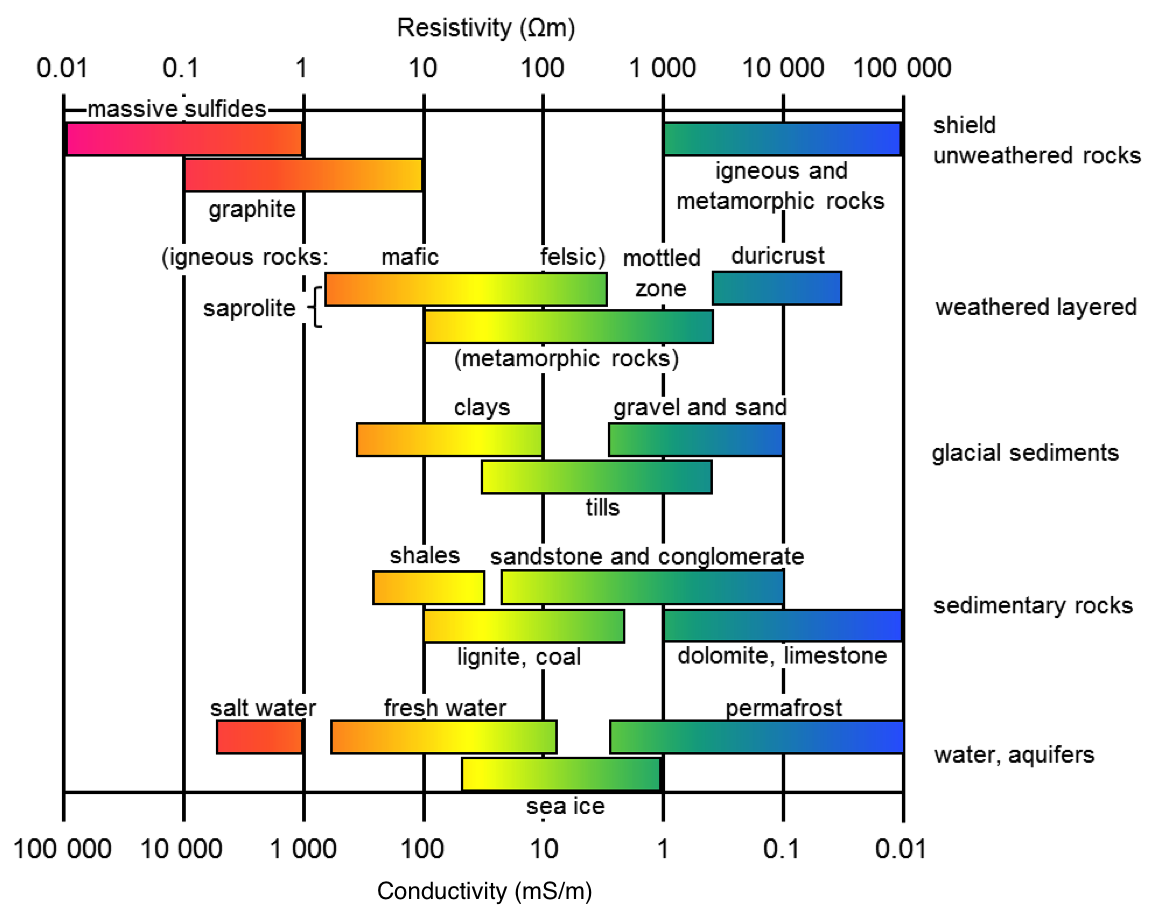# Conductivity¶

As part of the electromagnetics methods, DC resistivity is sensitive to electrical conductivity $$\sigma$$ (Siemens/meter). We sometimes work with the inverse of conductivity which is electrical resistivity $$\rho$$ which has units $$\Omega m$$ (ohm- meters). Electrical conductivity is a bulk property of material that characterizes the ease that current flows through the material when an electrical force is applied. Electric current (units of Amperes) quantifies the amount of charge that is moving by an observer in one second.

The electrical conductivity of Earth’s materials varies over many orders of magnitude. It depends upon many factors, including: rock type, porosity, connectivity of pores, nature of the fluid, and metallic content of the solid matrix. A very rough indication of the range of conductivity for rocks and minerals is presented in Fig. 161.Fig. 161 Resistivity/conductivity of common materials

Soils and rocks are composed mostly of silicate minerals, which are essentially insulators. Exceptions include magnetite, specular hematite, carbon, graphite, pyrite, and pyrrhotite. Therefore conduction is largely electrolytic, so conductivity depends mainly upon:

• porosity;

• hydraulic permeability, which describes how pores are interconnected;

• moisture content;

• concentration of dissolved electrolytes;

• temperature and phase of pore fluid;

• amount and composition of colloids (clay content).